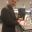# The perfect formula for mathsiness

It’s mid-January, which means it’s time for the tabloids to trot out their annual “this is the most miserable day of the whole year!” story — before they spend the rest of the year blaming immigration, youth and political correctness for problems they’ve spent the last year stoking up.

Ahem.

In any case, to pre-empt the inevitable “A Cambridge scientist has discovered the perfect formula for the perfect day of misery” angle, I thought I should give the perfect formula for the perfect formula in a nonsense mathematical story, bearing in mind Goldacre’s law:

All stories that claim to have found the perfect formula for anything are PR hogwash.

Here is the perfect formula for the mathsiness factor of a tabloid maths equation:

$MF = \frac{al \times mnoliv \times cs + di + mpf \sqrt{imn^3}}{(pra + sc)}+ul$

According to boffin Dr Colin Beveridge [hey! that’s me!], a maths tutor in Poole, the perfect formula for mathsy story has a large number of arbitrary letters ($a$) with unusually long names ($mnoliv$ is the maximum number of letters in a variable) and corporate sponsorship ($cs$, in pounds). The formula, commissioned by Flying Colours Maths, also rates stories by their dimensional inconsistency ($di$), mentions of the phrase ‘perfect fomula’ ($mpf$) and irrelevant mathematical notation ($imn$).

Counting against mathsiness are such irrelevancies as the number of peer-reviewed articles related to the story ($pra$ is typically 0) and scientific content ($sc$ is typically on the order of $10^{-7}$).

As it happens, this article has a mathsiness factor — or $MF$ — of around nearly three billion. I look forward to the Daily Mail’s attempts to beat this score over the coming year.

•#### Colin Beveridge

Colin Beveridge is the author of The Maths Behind and several other popular maths books. Based in Weymouth, Dorset, he divides his time between looking after his children and writing about maths.

### 5 Responses to “The perfect formula for mathsiness”

1.Ralph Hartley

Shouldn’t it also have many undefined variables?
Does “ul” incorporate that?.
No points for incmprehensible symbols?

•Christian Perfect
I hadn’t noticed $ul$ is unaccounted for! Extra plausibility. Can we sneak a $\gtreqless$ or two in there somehow? It’s my new favourite symbol.
•Colin Beveridge